Showing posts with label SharePoint Site Owner. Show all posts
Showing posts with label SharePoint Site Owner. Show all posts

3/16/2019

SharePoint Validation Formula Tip – Don't Use IF!

I often see questions about SharePoint validation formulas in the online forums that include IF statements to return True or False. Something like this:

=IF(  someCondition , True, False  )
=IF( Amount > 100, True, False )
=IF( AND( State = "OH", Amount>100 ), True, False )

The IF is simply not needed!

Simply replace this:
=IF( Amount > 100, True, False )
With this:
=Amount > 100

It's either greater than, or it is not. The greater than test returns a True or False all by itself. For that matter, the expression in the first parameter of an IF statement must return True or False!

Here's what it looks like in both Classic and Modern UI.

What if you need to reverse the True and the False result?

Use the NOT function to reverse the True/False value.
=NOT( Amount > 100 )

ANDs and ORs Return True or False

Simply replace this:
=IF( AND( State = "OH", Amount>100 ), True, False )
With this:
=AND( State = "OH", Amount>100 )

Two more examples…

If the state must be within the tri-state area you could write:
=IF( State="OH", True, IF( State="KY", True", IF( State="IN",True, False ) ) )
Or you could just write:
=OR( State="OH", State="KY", State="IN" )

While there are examples that require IF, you can solve most validations with a simple comparison, or the with the use of AND, OR and NOT.

=IF( AND( Amount>100, IF( State="OH", True, IF( State="KY", True", IF( State="IN",True, False ) ) ) ), True, False)

Yup… I have seen those. And, it can be replaced with:
=AND( Amount>100, OR( State="OH", State="KY", State="IN" ) )

And… book plug here… I have a lot more on validation formulas in my book!

10/30/2018

SharePoint Calculated Columns – Convert a Month Name to a Number

Here are three solutions for when you have a column with a three letter abbreviation of a month name, and you want the month number,

Each of the following assume that you have a "Single Line of Text" or "Choice" column named "Month".

A long IF statement:

IF([Month]="JAN",1,
IF([Month]="FEB",2,
IF([Month]="MAR",3,
IF([Month]="APR",4,
IF([Month]="MAY",5,
IF([Month]="JUN",6,
IF([Month]="JUL",7,
IF([Month]="AUG",8,
IF([Month]="SEP",9,
IF([Month]="OCT",10,
IF([Month]="NOV",11,
IF([Month]="DEC",12,
0
))))))))))))

Using the FIND() Function:

=(
FIND(
UPPER(D2),
"   ,JAN,FEB,MAR,APR,MAY,JUN,JUL,AUG,SEP,OCT,NOV,DEC")
) -1
) / 4

FIND returns the position of the matching text. "JAN" returns five. If we subtract one and then divide the FIND number by four, we get the month number.

Finding the Month Number Using Three or More Month Letters

=(
FIND(
UPPER(Month),
"         ,JANUARY  ,FEBRUARY ,MARCH    ,APRIL    ,MAY      ,JUNE     ,JULY     ,AUGUST   ,SEPTEMBER,OCTOBER  ,NOVEMBER ,DECEMBER "
)  -1
) / 10

This uses the same idea, but the month names are spelled out and are padded with spaces to make each one nine characters long. (Ten with the commas.)

Here’s the result: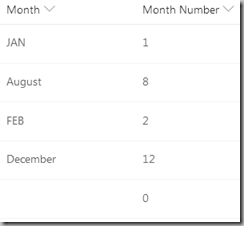Now available on Amazon!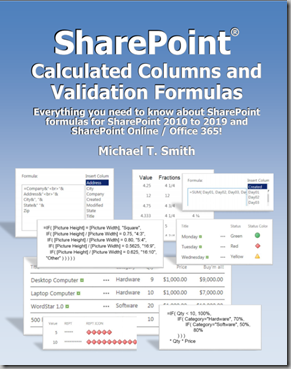10/15/2018

SharePoint Calculated Columns and Validation Formulas – The Book!

Available on Amazon!

It only took me a year… and it grew from a little booklet to over 200 pages… but its finally done!

Bought the book? Post any questions, bugs, typos and suggestions to this blog post!

Everything you need to know about SharePoint formulas for SharePoint 2010 to 2019 and SharePoint Online / Office 365!

This book is for the SharePoint “power user” who needs a better understanding of how SharePoint formulas work, where they don’t work, and how they are created. While at first glance SharePoint formulas appear to be simple calculations, they are both quite powerful and have weird limitations. In this book we will explore the basics of creating Calculated Columns and Validation formulas with some boring details, and over one hundred examples. We also explore workarounds for many of the limitations by using SharePoint Designer workflows and a few tricks!

Over 100 Examples!

A how-to book of formulas would not be too useful without a few examples. I've been collecting these for years. They've come from classroom questions, forum questions, and my own SharePoint sites. Now they are all in one place…

• · Over 60 Calculated Columns examples
• · Over 30 Column Validation examples
• · 11 List/Library Item Validation examples
(and every one of the Column Validation examples can be used here.)
• · 7 Calculated Column Default Values examples
• · 15 Workflow “workarounds” for things SharePoint formulas can’t do

Scroll on down for a complete list of the examples.

Who is this book for?

Anyone who creates and customizes lists and libraries. These include: SharePoint on premise Farm Administrators, Office 365 SharePoint administrators, Site Collection Administrators, Site Owners, Power users, and Developers.

Where are formulas used in SharePoint?

• Calculated column formulas derive data from other columns in the same list item. These can be simple calculations and text manipulations, or complex Excel-style formulas.
• Column validation formulas are used to create custom validations for user entered data. These can be used to make sure that "quantities" are not negative and vacation requests are for dates in the future.
• Column default formulas, while very limited, can auto-fill columns with a date 30 days in the future or a message based on the month or day of the week the item was added.
• List / Library validation formulas are used to check the values from multiple columns to verify the relationship between two or more columns. Examples include making sure a task start date is before the task end date, or to make sure an entry has a "price per pound" or a "price each", but not both.

Workflow Workarounds?

SharePoint formulas only work with a few column types while SharePoint workflows can access just about any column type. So, with a little workflow work your formulas can make these columns available to SharePoint formulas. There's over fifty pages of workflow workaround tips:

• Workaround for People and Groups
• Getting Data from a Person or Group Column
• Getting a User’s Manager
• Workaround for the SUBSTITUTE Function
• Workaround for Getting View Totals from Calculated Columns.
• Workaround for Adding Images to a Column
• Workaround for Multiple Lines of Text
• Workaround for Lookup Columns
• Lookup a Single Item (No checkboxes)
• Extract Several Lookup Columns into a Single Line of Text
• Workaround for Multivalued Choice Columns
• Counting Items Selected in a Multivalued Column
• Workaround for Managed Metadata columns
• Workaround for Single Valued Managed Metadata Columns
• Workaround for Multi Valued Managed Metadata Columns
• Workarounds for Attachments

• Read Me First
• Tips for Formulas
• Calculated Columns
• Calculated Column Examples
• Calculated Default Columns
• Column Validation Formulas
• Column Validation Examples
• List/Library Item Validation
• List/Library Item Validation Examples
• Other Forms of Validation
• Workflow Workarounds
• Error Messages

Examples:

Calculated Column Examples:·

·        Fun stuff that may not work for you:

o   Add HTML to a Calculated Column

o   Add icons or pictures to a Calculated Column

o   Building an Address (String Concatenation)

o   Create a Bar Chart Column

·        Columns for Views

o   View Filtering on a Calculated Column

o   Group by Year

o   Group by Month and Year

o   Group by Year Plus Month

o   Grouping on an Algorithm

·        Numbers

o   Adding Leading Zeros to a Number

o   Scientific Notation

o   Roman Numerals

·        The IF Function and Boolean Logic

o   Calculating a Discount using Nested IFs

o   Working Around Nested IF Limits

o   Convert from State Codes to State Names

o   ANDs and ORs - Approve if all approved, or reject if any one rejects

·        Test for values

o   Testing for a Range of Dates

o   Testing for Empty Columns

o   Testing for Errors

·        Summing and Counting Columns

o   Counting Yes/No Columns

o   Average

o   MIN and MAX

·        More on Numbers

o   Raise a Number to a Power

o   Rounding Numbers

·        Working with Text

o   Combining Text Columns

o   Display First Name and Last Name in a Single Column

o   Creating Title Capitalization

·        Return the Right Data Type!

·        Dates

o   Subtracting Dates

o   Finding a Date One Year in the Future

o   Change Date Formatting

o   Fiscal Year

o   Week Number

o   Day of the Year

o   First Day of the Current Month

o   First Day of the Next Month

o   Last Day of the Current Month

o   Last Day of the Previous Month

o   Last Day of the Next Month

o   Third Tuesday of a Month

o   Skipping Weekend Days

o   Next Workday “X” Days in the Future

o   Simple Solutions for 5, 10, 15 Working Days in the Future

o   Solution for Any Number of Working Days in the Future

o   Working Days

o   Number of Working Hours Between Two Dates

·        Formatting and Conversions

o   Formatting Numbers

o   Adding Text to Numbers

o   Adding Special Symbols (¥, £, etc.) to TEXT() Number Formats

o   Converting Numbers to Fractions

o   Financial Calculations

·        Random Numbers and Messages

o   Creating Random Numbers (Using NOW)

o   Creating Random Messages (using CHOOSE)

·        A Calculated Task Status

·        Great Circle Distance from Longitude and Latitude

·        Simplify a Workflow by Using a Calculated Column

Column Validation Examples

·        Limit time for user updates.

·        Boolean operations

o   Examples Using “OR”

o   Examples Using “AND”

o   Examples Using “AND” and “OR”

o   Examples Using “NOT”

·        Testing for Empty Columns

o   Yes/No

o   Dates

o   ISBLANK()

·        Working with Dates

o   Date Must be in the Future

o   Date Must be in the Future “x” Days

o   Date Must be in the Future “x” Days and not on a Weekend

o   Test Against a Specified Date

o   Date Must be Between Now and the End of the Current Year

o   Date Must be Within the Next 30 days

o   Date Must be the Last Day of the Month

o   Date Must be the First Day of the Month

o   Date Must be the Third Tuesday of the Month

o   Date Can Only be a Weekday

o   Date Can Only be Monday or Wednesday

o   Entered Date Must be for this Year

o   Date Must be Current Or Next Month Only

·        Working with Numbers

o   Testing for Numbers in Custom Increments

o   Limit the Number of Decimal Places Entered

·        Working with Text

o   Testing for Text Length

o   Testing for a Valid State/Province (or other code)

o   Test to Require an Uppercase or Lowercase Letter

·        Examples Using Pattern Matching

o   Must start with an "a" or "A" and the third character must be a "c" or "C"

o   Match a Phone Number Pattern of xxx-xxx-xxxx

o   Match a Phone Number Pattern of xxx-xxx-xxxx and Limit the Length

o   Match a Phone Number and Make Sure Only Digits Have Been Used

And many more!

9/06/2018

A Quick SharePoint Date Tip!

When you create a Date column you have the choice of Date and Date & Time.

Note the keyword “Format” in that option. Even if you select “Date Only”, your users can still type, or copy and paste, a date and a time and it will be stored as a date and time. But… only the date will be displayed.

Times are a Fraction of a Date

Times are represented as parts of a day.

Converting a Date and Time to a Date

As you saw above, SharePoint dates are always dates and times. Even if you set a column to be “Date”, users can still type, or copy and paste, Date and Time values. Columns formatted as “Date” may display as just a date, but they will still be filtered and calculated as a Date and Time.

If you are calculating the number of days between two dates you might write “[Date2]-[Date1]”, which will produce the expected result if both dates are true Date values. If Date2 is “1/16/2018 6:00 AM” and formatted as “1/16/2018”, and Date1 is “1/14/2018” subtracting the two will return 2.25 days, not the hoped for 2 days.

There are several ways of converting a Date and Time to a Date:

• Use the DATE function:
DATE( YEAR( [SomeDate] ), MONTH(( [SomeDate] ), DAY( [SomeDate] ) )
• Use the ROUND function:
ROUND( [SomeDate], 0) (This will round up or down depending on the value)
• Use the FLOOR (round down) function:
FLOOR( [SomeDate], 1)
• Use the CEILING (round up) function:
CEILING( [SomeDate], 1)
• Use the INT function (which also rounds down):
INT( [SomeDate])

I prefer the INT as it is the least typing and I most often need to round down to remove the fractional part of a Date and Tim

4/20/2018

The Mysterious Semicolon in SharePoint Search

Discoveries:

• A semicolon in a search is the same as the keyword "AND".
• You cannot uniquely find text that contains a semicolon. (such as ABC;DEF)
• A semicolon used as data in a checkbox enabled Choice column causes data and search problems.

If you have more info, or know of an escape character for “;” please post a note to this article!

";" equals "AND"?

If I were to search a SharePoint list for "111 AND 222", I would find items where "111" might be in one column and "222" might be in another, or both are two words in the same column. I was trying to find data that looked like this, "111;222", and was getting wrong matches. At first I thought the semicolon was behaving as a wildcard, and that would have been really cool as SharePoint does not support anything other than an "*" at the end of a word.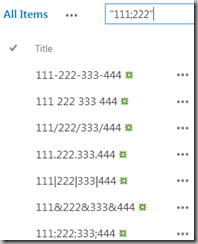From testing, it appears that a semicolon is identical to the keyword "AND". In the example below, note that all of the sample text entries are using "word delimiters" that SharePoint recognizes as search word breakers. As "data" a semicolon is treated as "white space" or a word breaker, and is ignored by search!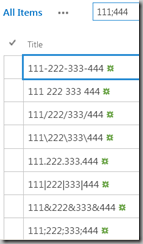Here is a search for "111" AND "44" and note that no items were found.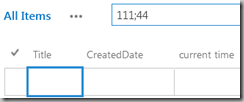You can't uniquely search for a semicolon!

If your data contains symbols in the middle of text, you will have a problem when searching for text that contains a semicolon. In the example below, note that the search for "555;777" also finds other items.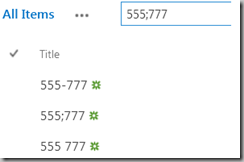The addition of quotes will solve this problem for other special characters, but not the semicolon.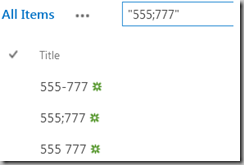Semicolons and Choice Columns

A discussion of the semicolon would not be complete if we don't talk about what it does to a Choice column!

Consider Choice column with these choices: (third choice has a semicolon)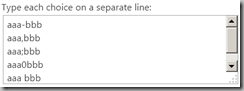And for this Choice column we selected Checkboxes.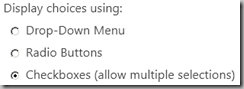Now let's select some data. Notice the display when I select two items in a QuickEdit view. The semicolon is part of one item's data, and is also there as a delimiter between items.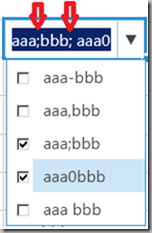When I click off of that row, note that the two selected items are being treated as three items!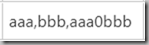When I attempt to edit that column again, the item with the semicolons has been lost because there are no "aaa" or "bbb" items in the list.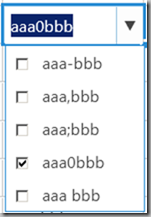So…

• Don't put semicolons in your data if you plan to search for it.
• Don't put semicolons in multi-choice (Checkbox) columns.

4/07/2018

SharePoint TEXT() Function Bug for Scientific Notation

In Excel you can use the TEXT function to display very large and very small numbers in scientific notation.

TEXT([Number],"0.00E+00")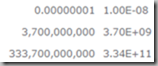Due to a bug in SharePoint Calculated Columns, the TEXT function adds some extra zeros to the end of the formatted number.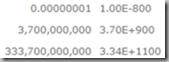Here’s a workaround…

Assuming you want the equivalent of TEXT([Number],"0.00E+00")

=LEFT(TEXT(Number,"0.00E+00"),6) &
TEXT(MID(TEXT(Number,"0.00E+00"),7,LEN(TEXT(Number,"0.00E+00"))-8),"00")

This part:

LEFT(TEXT(Number,"0.00E+00"),6)

Pulls off the "3.70E+".

This part:

MID(TEXT(Number,"0.00E+00"),7,LEN(TEXT(Number,"0.00E+00"))-8)

Pulls off the "9" and formats it as "09”.

To change the format, adjust the number of zeros in the obvious places, and adjust the "6", "7" and "8" as needed. Here two examples to help you figure out which numbers to change.

For the equivalent of TEXT([Number],"0.00E+000")

=LEFT(TEXT(Number,"0.00E+000"),6) &
TEXT(MID(TEXT(Number,"0.00E+000"),7,LEN(TEXT(Number,"0.00E+000"))-9),"000")

For the equivalent of TEXT([Number],"0.000E+00")

=LEFT(TEXT(Number,"0.000E+00"),7) &
TEXT(MID(TEXT(Number,"0.000E+00"),8,LEN(TEXT(Number,"0.000E+00"))-9),"00")

Here the "0.00E+00", "0.00E+000" and "0.000E+00" results.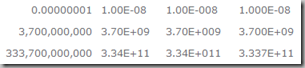I just listed this as a bug. If you would like to vote for a fix, go here: https://sharepoint.uservoice.com/forums/329214-sites-and-collaboration/suggestions/33878629-fix-text-function-bug-for-scientific-notation

As the feature has minimal usage, and has been there forever, I don't expect too much... ;-)

.

3/29/2018

Shorter SharePoint Calculated Column OR Statements

Tested in SharePoint 2010, 2013, 2016 and Online.

Array Constants

Excel will often let you use an array of values where you would normally use a range of cells.

The Excel version:

=VLOOKUP( [StatusCode],
{"a","Active";"i","Inactive";"c","Closed"}, , 2, 0 )

The array constant part of the formula is from “{“ to “}” and represents a two dimensional table. The Excel range equivalent to this array looks like this:

But…

While that is kind of cool, it does not always work in SharePoint Calculated Columns. One place it does work is with an OR function where it works much like the “IN” keyword in other technologies. The following two functions are equivalent:

=IF( OR( StateCode="OH", StateCode="KY", StateCode="IN", StateCode="IL" ),
"Central Region", "Other" ) =IF( OR( StateCode = {"OH","KY","IN","IL"} ), "Central Region", "Other")

(If you see this anywhere other than at TechTrainingNotes.blogspot.com, it was stolen and used without permission!)

Both of the above produce this result:

Pretty cool!

3/26/2018

Adding Fractions (1/4) to SharePoint Calculated Columns

(The following works SharePoint 2010, 2013, 2016, SharePoint Online, and most likely, 2019!)

0, 1/8, 1/4, 3/8, 1/2, 5/8, 3/4, and 7/8

This is a sample from my soon to be published book “SharePoint Calculated Columns and Validation Formulas”. If you would like to see a lot more about what you can do with Calculated Columns, and maybe win a free book, attend my session at SharePoint Cincy 2018 on April 10th!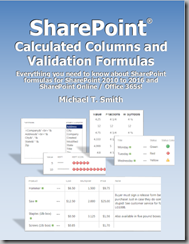In Excel you can get fractions using =TEXT(yourNumberColumn,"# ?/?"):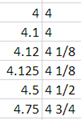The same function in SharePoint will round off the number, and for each of the above values, return 5. If you need fractions then you will need to write your own function to create them. Below we have several versions of this function depending on the fractions and formatting needed.

Function to return the fractions 0, 1/4, 1/2, and 3/4

Note: Read to the end to see a shorter version that uses CHOOSE instead of nested IFs.

Just a couple of nested IFs…

=IF( yourNumberColumn - INT(yourNumberColumn) = 0.25,
INT( yourNumberColumn) & " 1/4",
IF( yourNumberColumn - INT(yourNumberColumn) = 0.5,
INT( yourNumberColumn) & " 1/2",
IF( yourNumberColumn - INT(yourNumberColumn) = 0.75,
INT( yourNumberColumn) & " 3/4",
INT(yourNumberColumn) ) ) )

The above formula only works if the entered values end in exactly 0, .25, .5 and .75. If you need your function to round off to the nearest 1/4th, replace the first value in each IF with:

ROUND( yourNumberColumn * 4, 0 ) / 4

=IF( ROUND( yourNumberColumn*4, 0 ) / 4 - INT(yourNumberColumn) = 0.25,
INT(yourNumberColumn) & " 1/4",
IF( ROUND(yourNumberColumn * 4, 0 ) / 4 - INT(yourNumberColumn) = 0.5,
INT(yourNumberColumn) & " 1/2",
IF( ROUND(yourNumberColumn * 4 , 0 ) / 4 - INT(yourNumberColumn) = 0.75,
INT( yourNumberColumn) & " 3/4",
ROUND( yourNumberColumn,0) ) ) )

If you would like to see ¼ instead of 1/4 then use the CHAR function to get those symbols. While the ¼, ½, and ¾ characters are available, 1/3 and 1/8 are not.

• Replace " 1/4" with " " & CHAR(188)
• Replace " 1/2" with " " & CHAR(189)
• Replace " 3/4" with " " & CHAR(190)
=IF( ROUND( yourNumberColumn * 4, 0 ) / 4 - INT(yourNumberColumn) = 0.25,
INT(yourNumberColumn) & " " & CHAR(188),
IF( ROUND(yourNumberColumn * 4, 0 ) / 4 - INT(yourNumberColumn) = 0.5,
INT(yourNumberColumn) & " " & CHAR(189),
IF( ROUND(yourNumberColumn * 4, 0 ) / 4  -INT(yourNumberColumn)=0.75,
INT( yourNumberColumn) & " " & CHAR(190),
ROUND( yourNumberColumn, 0 ) ) ) )

Function to return the fractions 0, 1/8, 1/4, 3/8, 1/2, 5/8, 3/4, and 7/8

To add support for eighths we need to add four more IFs. If we change the number used in our rounding code from 4 to 8, and add a few lines, then web can display values to the nearest eighth.

=IF( ROUND(yourNumberColumn * 8, 0 ) / 8 - INT(yourNumberColumn) = 0.125,
INT(yourNumberColumn) & " 1/8",
IF( ROUND(yourNumberColumn * 8, 0 ) / 8 - INT(yourNumberColumn) = 0.25,
INT(yourNumberColumn) & " 1/4",
IF( ROUND(yourNumberColumn * 8, 0 ) / 8 - INT(yourNumberColumn) = 0.375,
INT(yourNumberColumn) & " 3/8",
IF( ROUND(yourNumberColumn * 8, 0 ) / 8 - INT(yourNumberColumn) = 0.5,
INT(yourNumberColumn) & " 1/2",
IF( ROUND(yourNumberColumn * 8, 0 ) / 8 - INT(yourNumberColumn) = 0.625,
INT(yourNumberColumn) & " 5/8",
IF( ROUND(yourNumberColumn * 8, 0 ) / 8 - INT(yourNumberColumn) = 0.75,
INT(yourNumberColumn) & " 3/4",
IF( ROUND(yourNumberColumn * 8, 0 ) / 8 - INT(yourNumberColumn) = 0.875,
INT(yourNumberColumn) & " 7/8",
ROUND(yourNumberColumn, 0 ) ) ) ) ) ) ) )

Now we have fractions to the nearest 1/8th.

A Much Shorter Version that uses CHOOSE

Here is a much shorter version of a formula to display a number as a fraction:

=ROUNDDOWN( yourNumberColumn + 0.062499, 0 ) &
CHOOSE( ( ROUND( yourNumberColumn * 8, 0 ) / 8
- INT(yourNumberColumn) ) * 8 + 1,
""," 1/8"," 1/4"," 3/8"," 1/2", " 5/8", " 3/4", " 7/8",""
)

This uses CHOOSE to pick the text to display for “less than 1/8th”, “1/8th” … “7/8th” and “more than 7/8th”. (Nine possible values.)

To get the fraction:

1. Round the number to the nearest 1/8th:
( ROUND( yourNumberColumn * 8, 0 ) / 8 - INT(yourNumberColumn) ) * 8
2. And as CHOOSE starts with 1 and not 0, add one to the result:
( ROUND( yourNumberColumn * 8, 0 ) / 8 - INT(yourNumberColumn) ) * 8 + 1
3. Use CHOOSE to pick the text:
CHOOSE( … , ""," 1/8"," 1/4"," 3/8"," 1/2", " 5/8", " 3/4", " 7/8","")
4. Concatenate that to the rounded down number:
ROUNDDOWN( yourNumberColumn + 0.062499, 0 ) & …
The “+ 0.062499” is added to deal with the last 1/16th after “7/8th” so we round up to the next higher number..

3/08/2018

Adding Roman Numerals to SharePoint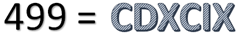In a Calculated Column

Assuming an integer in a numeric column named the "TheNumber", just add this equation to a Calculated Column:

=ROMAN(TheNumber)

You can also select alternate formats:

In a Page, Content Editor Web Part or a Rich Text Multiple Lines of Text column.

The Rich Text ribbon offers buttons to create bullets and numbers, but does not have one to select the other list formatting options. With a little HTML edit you can get any of the HTML list styles.

1. Create the numbered list as usual. (1., 2., 3....)
2. Click the "Edit Source" button in the ribbon.
3. Find the <ol> tag and change it to <ol type="I">. (Use a lower case "i" for lower case Roman numerals.)
4. Click OK.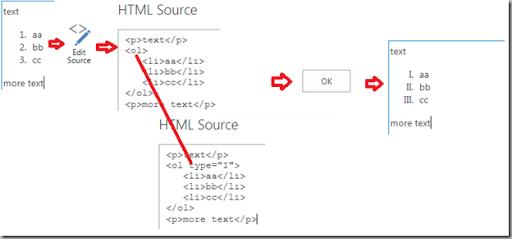When starting with a numbered list you can choose any of the HTML ordered lists (<OL>) types:

• type = "1" – numbers
• type = "A" – Uppercase letters: A, B, C
• type ="a" – Lowercase letters:  a, b, c
• type – "I" – Uppercase Roman numerals: I, II, III
• type = "I" – Lowercase Roman numerals:  i, ii, iii

When starting with a bulleted list you can choose any of the HTML unordered lists (<UL>) types:

• disc
• circle
• square
• none

You can also use your own custom images. See: https://www.w3schools.com/cssref/pr_list-style-image.asp

.

3/07/2018

A SharePoint Calculated Column for all 50 States (workarounds for nested IF limits!)

Just in case you might ever need a formula to convert state abbreviations into state names…

• You will need 50 nested IFs,
• but SharePoint 2007 and 2010 only allows 7, and 2013 and later only allows 19.
• You will need a little more than 1300 characters in the formula,
• but SharePoint 2007 and 2010 only allow 1024. (2013 and later are around one billion!)

The trick for the IFs is to only nest 19 at a time and return a state name, or an empty string (""), and then concatenate another 19 nested IFs that return a state name, or an empty string… repeat until done! If you are using 2007 or 2010, then nest 7 at a time, and then concatenate another 7.

But what about the 2007 and 2010 1024 character limit? Renaming the "state" column to just one letter brings the formula down to 1111 characters, but that's still more than the 1024 allowed. Solution? Three Calculated columns. The first has the formulas for the first 25 states (in multiple IF nestings of 7 or less) that returns a state name or an empty string, The second has the next 25 states and returns a state name or an empty string. The third just concatenates the first two columns.

Here's the formula for SharePoint 2013 and later for a column named "State":

if(State="AL","Alabama",
if(State="AZ","Arizona",
if(State="AR","Arkansas",
if(State="CA","California",
if(State="CT","Connecticut",
if(State="DE","Delaware",
if(State="FL","Florida",
if(State="GA","Georgia",
if(State="HI","Hawaii",
if(State="ID","Idaho",
if(State="IL","Illinois",
if(State="IN","Indiana",
if(State="IA","Iowa",
if(State="KS","Kansas",
if(State="KY","Kentucky",
if(State="LA","Louisiana",
if(State="ME","Maine",""))))))))))))))))))) &
if(State="MD","Maryland",
if(State="MA","Massachusetts",
if(State="MI","Michigan",
if(State="MN","Minnesota",
if(State="MS","Mississippi",
if(State="MO","Missouri",
if(State="MT","Montana",
if(State="NH","New Hampshire",
if(State="NJ","New Jersey",
if(State="NM","New Mexico",
if(State="NY","New York",
if(State="NC","North Carolina",
if(State="ND","North Dakota",
if(State="OH","Ohio",
if(State="OK","Oklahoma",
if(State="OR","Oregon",
if(State="PA","Pennsylvania",""))))))))))))))))))) &
if(State="RI","Rhode Island",
if(State="SC","South Carolina",
if(State="SD","South Dakota",
if(State="TN","Tennessee",
if(State="TX","Texas",
if(State="UT","Utah",
if(State="VT","Vermont",
if(State="VA","Virginia",
if(State="WA","Washington",
if(State="WV","West Virginia",
if(State="WI","Wisconsin","")))))))))))

Note to spammers!

Spammers, don't waste your time... all posts are moderated. If your comment includes unrelated links, is advertising, or just pure spam, it will never be seen.